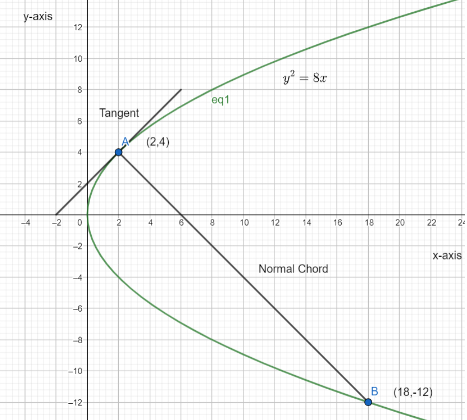Courses
Courses for Kids
Free study material
Offline Centres
MoreLast updated date: 30th Nov 2023
Total views: 277.5k
Views today: 4.77k

# Show that the normal to the parabola ${{y}^{2}}=8x$ at $\left( 2,4 \right)$ cuts the parabola again at $\left( 18,-12 \right)$ also find the length of normal chord.Verified
277.5k+ views
Hint: To show that the normal of the given parabola at $\left( 2,4 \right)$ cuts the parabola again at $\left( 18,-12 \right)$ we will use the slope of the tangent formula. Firstly we will find the slope of the tangent by differentiating the given equation with respect to $x$ and equate the point which cuts the parabola. Then by using it we will find the slope of normal and get the equation of normal. Finally we will obtain the obtained equation in the parabola equation to prove our point. For finding the length of a normal chord we will use the distance formula.

Complete step-by-step solution:
The parabola given is:
${{y}^{2}}=8x$…..$\left( 1 \right)$
Firstly we will find the slope of tangent by differentiating the above equation with respect to $x$ as follows:
\begin{align} & \dfrac{d}{dx}\left( {{y}^{2}} \right)=\dfrac{d}{dx}\left( 8x \right) \\ & \Rightarrow 2y\dfrac{dy}{dx}=8 \\ & \Rightarrow \dfrac{dy}{dx}=\dfrac{8}{2y} \\ & \therefore \dfrac{dy}{dx}=\dfrac{4}{y} \\ \end{align}
So the parabola is cut at point $\left( 2,4 \right)$ so slope of tangent will be equating the point in above value as:
\begin{align} & \dfrac{dy}{dx}=\dfrac{4}{4} \\ & \therefore \dfrac{dy}{dx}=1 \\ \end{align}
Slope of tangent is 1.
Now as we know slope of normal is negative inverse of slope of tangent.
Slope of Normal is $-1$.
Now we will find the Equation of Normal at point $\left( 2,4 \right)$ with slope $-1$ as follows:
So
\begin{align} & \left( {{x}_{1}},{{y}_{1}} \right)=\left( 2,4 \right) \\ & m=-1 \\ \end{align}
Equating above value in formula $y-{{y}_{1}}=m\left( x-{{x}_{1}} \right)$ we get,
\begin{align} & y-4=-1\left( x-2 \right) \\ & \Rightarrow y-4=-x+2 \\ & \Rightarrow x=2+4-y \\ \end{align}
$\therefore x=6-y$…..$\left( 2 \right)$
As normal touches the equation (1) it will satisfy it also.
So equating value from equation (2) in equation (1) we get,
\begin{align} & {{y}^{2}}=8\left( 6-y \right) \\ & \Rightarrow {{y}^{2}}+8y-48=0 \\ & \Rightarrow {{y}^{2}}+12y-4y-48=0 \\ & \Rightarrow y\left( y+12 \right)-4\left( y+12 \right)=0 \\ \end{align}
$\Rightarrow \left( y-4 \right)\left( y+12 \right)=0$
$\Rightarrow y=4$, $y=-12$
We already know that the normal intersects at $\left( 2,4 \right)$.
So put $y=-12$ in equation (2) we get,
\begin{align} & x=6-\left( -12 \right) \\ & \Rightarrow x=6+12 \\ & \therefore x=18 \\ \end{align}
So normal cut the parabola at $\left( 18,-12 \right)$
Next we have to find the length of the normal chord.
So the point we have is $\left( 2,4 \right)$ and $\left( 18,-12 \right)$
Using distance formula we get,
Length of normal chord $=\sqrt{{{\left( 18-2 \right)}^{2}}+{{\left( -12-4 \right)}^{2}}}$
Length of normal chord $=\sqrt{256+256}$
Length of normal chord $=\sqrt{512}$
Length of normal chord $=16\sqrt{2}$
Hence normal to the parabola ${{y}^{2}}=8x$ at $\left( 2,4 \right)$ cuts the parabola again at $\left( 18,-12 \right)$ and length of normal chord is $16\sqrt{2}$.Note: A parabola is a curve where any point is at an equal distance from a fixed point and a fixed straight line. We can also see graphically that the two points are the normal to the parabola in the graph below. Also if the point lies in the parabola it will always satisfy the equation of the parabola.